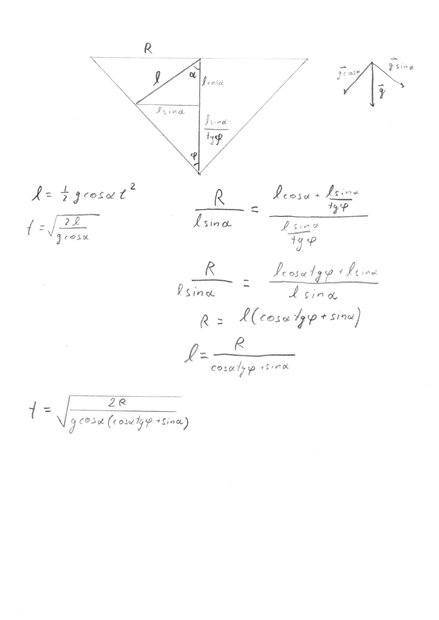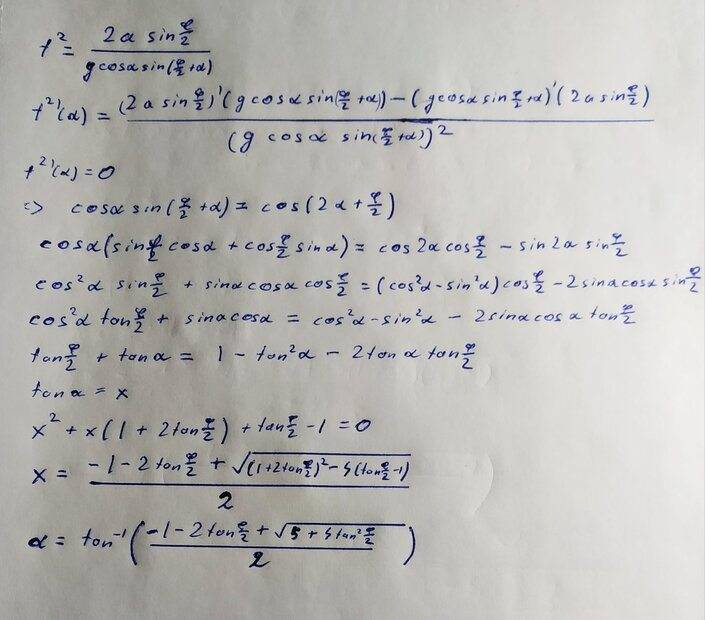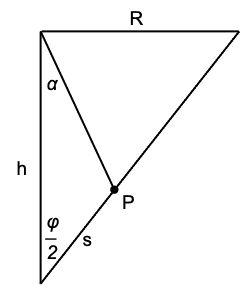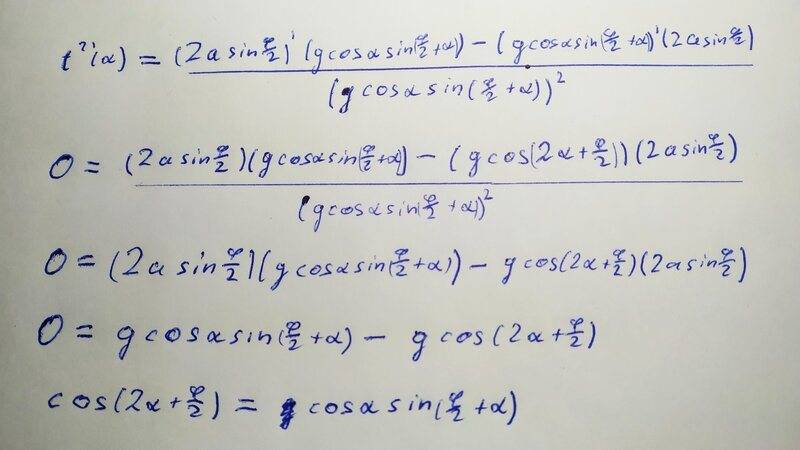# String in cone problem

bowes78
Homework Statement:
A hollow cone is placed on the ground like shown in example. Inside a cone there is string attached to base center. In the string there is a small bead. What must the angle between string and vertical be, so bead reaches cone surface fastest? (You can stretch the string). Cone base is parallel to the ground and cone has angle φ. String frictionless.
Relevant Equations:
kinematic eqs
trig identites
I tried to come up with expression of time in terms of φ, α, and some constant value of cone size that does not depend on angle (I used R as cone radius). I though I could use that expression to see at what angle time is going to minimum, but I came up with expression from which I can't make a conclusion. I believe there is another solution method, or maybe there are some useful trig identities, but I was unable to find it.
Can someone help me out? Thanks

Angle φ is marked badly at my solution, but that doesn't change anything in particular.#### Attachments

Homework Helper
Gold Member
Note that the angle you call ##\varphi## is half the angle given as ##\varphi## in the diagram.

My first approach would be to use the law of sines for the triangle that has angles ##\alpha##, ##\varphi/2## and ##\pi-(\alpha+\varphi/2).## Then minimize ##t^2## with respect to ##\alpha.##

•SammyS
bowes78
Note that the angle you call ##\varphi## is half the angle given as ##\varphi## in the diagram.

My first approach would be to use the law of sines for the triangle that has angles ##\alpha##, ##\varphi/2## and ##\pi-(\alpha+\varphi/2).## Then minimize ##t^2## with respect to ##\alpha.##
So I get t^2=(2 a sin(φ/2))/(g cosα sin(φ/2+α)), a is length OC. How can I minimize it to find value of α?

Homework Helper
Gold Member
2022 Award
So I get t^2=(2 a sin(φ/2))/(g cosα sin(φ/2+α)), a is length OC. How can I minimize it to find value of α?
How do you usually find a minimum of a function wrt its independent variable?

bowes78
How do you usually find a minimum of a function wrt its independent variable?
So after differentiation with respect to α, setting t=0, I get α=90-(φ/2). Could this be the answer?

Homework Helper
Gold Member
2022 Award
So after differentiation with respect to α, setting t=0, I get α=90-(φ/2). Could this be the answer?
Consider what that looks like in your diagram. It would mean the string meets the cone at a right angle. It is easy to show that the answer must have the string meet the cone somewhat lower.

bowes78
Consider what that looks like in your diagram. It would mean the string meets the cone at a right angle. It is easy to show that the answer must have the string meet the cone somewhat lower.
Yes, previous solution had a mistake. This time I tried to do something like this, but that seems to me that it doesn't make sense too. Because if we set (φ/2)=0, then α=32, which doesn't look right.Homework Helper
Gold Member
What about the work before what you posted? Specifically, what does ##a## stand for in the first equation giving ##t^2##?

bowes78
What about the work before what you posted? Specifically, what does ##a## stand for in the first equation giving ##t^2##?
I just picked there wrong trigonometry formulas, so I got wrong answer. As I mentioned before, a is length OC in the triangle. I needed that for law of sines.

Homework Helper
Gold Member
The law of sines is always two equations. In this case is $$\frac{s}{\sin\alpha} =\frac{\frac{1}{2}gt^2\cos\alpha}{\sin(\varphi/2)} =\frac{h}{\sin(\alpha+\varphi/2)}.$$
Did you use both? The first equation involving segment ##s## is needed to ensure that the point of intersection P is on the hypotenuse at time ##t##.

It's probably easier and more straightforward to write equations for the position of the bead, ##x(t)## and ##y(t),## find the intersection with the hypotenuse and then minimize.Homework Helper
Gold Member
2022 Award
Yes, previous solution had a mistake. This time I tried to do something like this, but that seems to me that it doesn't make sense too. Because if we set (φ/2)=0, then α=32, which doesn't look right.
Not sure how you got the line following "=>". Please post the details of that step.
I assume you realise it is only necessary to maximise the denominator in the expression for ##t^2## since the numerator is constant.

bowes78
The law of sines is always two equations. In this case is $$\frac{s}{\sin\alpha} =\frac{\frac{1}{2}gt^2\cos\alpha}{\sin(\varphi/2)} =\frac{h}{\sin(\alpha+\varphi/2)}.$$
Did you use both? The first equation involving segment ##s## is needed to ensure that the point of intersection P is on the hypotenuse at time ##t##.

It's probably easier and more straightforward to write equations for the position of the bead, ##x(t)## and ##x(t)## find the intersection with the hypotenuse and then minimize.
View attachment 318945
No, I used only $$\frac{h}{sin(\alpha+\varphi/2)}=\frac{\frac{1}{2}gt^{2}cos\alpha }{sin(\varphi/2) }.$$

I think I'm getting your idea, but how I should write ##x(t)## equation? I suppose that $$y(t)=\frac{1}{2}gt^{2}.$$
Or it should be like $$y(t)=\frac{1}{2}gcos^{2}\alpha t^{2}$$
$$x(t)=\frac{1}{2}gsin\alpha cos\alpha t^{2}$$
Because string length is $${\frac{1}{2}gcos\alpha}t^{2}$$

bowes78
Not sure how you got the line following "=>". Please post the details of that step.
I assume you realise it is only necessary to maximise the denominator in the expression for ##t^2## since the numerator is constant.Homework Helper
Gold Member
2022 Award
a and φ are constants, so what is the derivative of ##2a\sin(\phi/2)##?

bowes78
a and φ are constants, so what is the derivative of ##2a\sin(\phi/2)##?
I see... It should be zero, but whole equation then disappears.

Homework Helper
Gold Member
2022 Award
I see... It should be zero, but whole equation then disappears.
Does it? What do you have left?

bowes78
Does it? What do you have left?
Well, should I solve it like that?
$$-gcos(2\alpha+\varphi /2)(2asin(\varphi/2))=0$$
$$cos(2\alpha+\varphi /2)=0$$
$$\alpha =\frac{90^{\circ}-\varphi /2 }{2}$$

Homework Helper
Gold Member
2022 Award
Well, should I solve it like that?
$$-gcos(2\alpha+\varphi /2)(2asin(\varphi/2))=0$$
$$cos(2\alpha+\varphi /2)=0$$
$$\alpha =\frac{90^{\circ}-\varphi /2 }{2}$$
Right.

bowes78
Right.
Thanks!